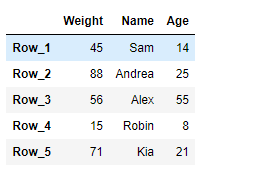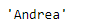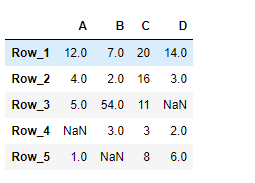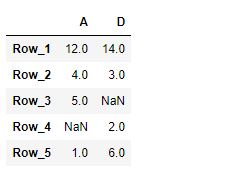GeeksforGeeks App
Open AppBrowser
Continue

# Pandas DataFrame.loc[] Method

Pandas DataFrame is a two-dimensional size-mutable, potentially heterogeneous tabular data structure with labeled axes (rows and columns). Arithmetic operations align on both row and column labels. It can be thought of as a dict-like container for Series objects. This is the primary data structure of the Pandas

## Pandas DataFrame loc[] Syntax

Pandas DataFrame.loc attribute access a group of rows and columns by label(s) or a boolean array in the given Pandas DataFrame.

Syntax: DataFrame.loc

Parameter : None

Returns : Scalar, Series, DataFrame

## Pandas DataFrame loc Property

Example 1: Use DataFrame.loc attribute to access a particular cell in the given Pandas Dataframe using the index and column labels.

## Python3

 `# importing pandas as pd``import` `pandas as pd` `# Creating the DataFrame``df ``=` `pd.DataFrame({``'Weight'``:[``45``, ``88``, ``56``, ``15``, ``71``],``                ``'Name'``:[``'Sam'``, ``'Andrea'``, ``'Alex'``, ``'Robin'``, ``'Kia'``],``                ``'Age'``:[``14``, ``25``, ``55``, ``8``, ``21``]})` `# Create the index``index_ ``=` `[``'Row_1'``, ``'Row_2'``, ``'Row_3'``, ``'Row_4'``, ``'Row_5'``]` `# Set the index``df.index ``=` `index_` `# Print the DataFrame``print``(df)`

Output:Now we will use DataFrame.loc attribute to return the value present in the ‘Name’ column corresponding to the ‘Row_2’ label.

## Python3

 `# return the value``result ``=` `df.loc[``'Row_2'``, ``'Name'``]` `# Print the result``print``(result)`

Output:

Here, the DataFrame.loc attribute has successfully returned the value present at the desired location in the given DataFrame.Example 2: Use DataFrame.loc attribute to return two of the column in the given Dataframe.

## Python3

 `# importing pandas as pd``import` `pandas as pd` `# Creating the DataFrame``df ``=` `pd.DataFrame({``"A"``:[``12``, ``4``, ``5``, ``None``, ``1``],``                ``"B"``:[``7``, ``2``, ``54``, ``3``, ``None``],``                ``"C"``:[``20``, ``16``, ``11``, ``3``, ``8``],``                ``"D"``:[``14``, ``3``, ``None``, ``2``, ``6``]})` `# Create the index``index_ ``=` `[``'Row_1'``, ``'Row_2'``, ``'Row_3'``, ``'Row_4'``, ``'Row_5'``]` `# Set the index``df.index ``=` `index_` `# Print the DataFrame``print``(df)`

Output:Now we will use DataFrame.loc attribute to return the values present in the ‘A’ and ‘D’ columns of the Dataframe.

## Python3

 `# return the values.``result ``=` `df.loc[:, [``'A'``, ``'D'``]]` `# Print the result``print``(result)`

Output :As we can see in the output, the DataFrame.loc attribute has successfully returned the desired columns of the Dataframe.

My Personal Notes arrow_drop_up